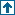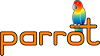parrotcode: Lexical Ops Contents | Tests

# NAMEt/op/lexicals.t - Lexical Ops

# SYNOPSIS`    % prove t/op/lexicals.t`

# DESCRIPTIONTests various lexical scratchpad operations, as described in PDD20.

```  ;;; Indicate that the computation has failed, and that the program
;;; should try another path.  We rebind this variable as needed.
(define fail
(lambda () (error "Program failed")))

;;; Choose an arbitrary value and return it, with backtracking.
;;; You are not expected to understand this.
(define (choose . all-choices)
(let ((old-fail fail))
(call-with-current-continuation
(lambda (continuation)
(define (try choices)
(if (null? choices)
(begin
(set! fail old-fail)
(fail))
(begin
(set! fail
(lambda () (continuation (try (cdr choices)))))
(car choices))))
(try all-choices)))))

;;; Find two numbers with a product of 15.
(let ((x (choose 1 3 5))
(y (choose 1 5 9)))
(for-each display `("Trying " ,x " and " ,y #\newline))
(unless (= (* x y) 15)
(fail))
(for-each display `("Found " ,x " * " ,y " = 15" #\newline)))```